﻿ SR Equations without Constant One-Way Speed of Light

SR Equations without Constant One-Way Speed of Light

Zhiliang Cao, Henry Gu CaoOPEN ACCESSPEER-REVIEWED

SR Equations without Constant One-Way Speed of Light

Zhiliang Cao1,, Henry Gu Cao2

1J.P.Morgan Chase

2Deerfield High School, Deerfield

Abstract

A constant one-way light speed is essential for the Theory of Relativity. This detailed study examines two postulates of Special Relativity and concludes that the claim of “constant one-way speed of light” contradicts itself. The equations of Special Relativity are foundations of many physics theories. The findings on controversial Relativity postulates shake the foundation of these theories. Fortunately, equations similar to Special Relativity equations can be derived, assuming that two-way light speed is constant, without the use of Special Relativity Postulates. These new equations provide a better foundation that is compatible with the correct existing physics theories. There is no threat of invalidating all existing physics theories, only incorrect ones. Instead, we can take a new look at some fundamental questions shared among physicists.

At a glance: Figures

12
Prev Next

• Cao, Zhiliang, and Henry Gu Cao. "SR Equations without Constant One-Way Speed of Light." International Journal of Physics 1.5 (2013): 106-109.
• Cao, Z. , & Cao, H. G. (2013). SR Equations without Constant One-Way Speed of Light. International Journal of Physics, 1(5), 106-109.
• Cao, Zhiliang, and Henry Gu Cao. "SR Equations without Constant One-Way Speed of Light." International Journal of Physics 1, no. 5 (2013): 106-109.

 Import into BibTeX Import into EndNote Import into RefMan Import into RefWorks

1. Introduction

Special relativity (e.g. [1-13]) is a theory about the structure of spacetime. It was introduced in Einstein's 1905 paper "On the Electrodynamics of Moving Bodies". Special relativity is based on two postulates which are contradictory in classical mechanics:

1. The laws of physics are the same for all observers in uniform motion relative to one another (principle of relativity).

2. The speed of light in a vacuum is the same for all observers, regardless of their relative motion or of the motion of the source of the light (Figure 1).

First, this paper studies the two postulates of Special Relativity and finds some contradictions. It mathematically proves that the “constant one-way speed of light” (e.g. [13-40]) made in second relativity claim is invalid.

Next, the paper derives relativity equations under assumption that aether exists. The new equations are similar to Relativity Equations, but the meanings are different.

2. Relativity Postulates

2.1. Relativity Postulates

Based on the second Relativity claim:

The speed of light in a vacuum is the same for all observers, regardless of their relative motion or the motion of the source of the light.

There are three main scenarios:

1. Two moving light sources with different speeds and one observer.

2. One light source and two observers with different moving speeds.

3. Two moving light sources and two observers with different moving speed.

Relativity only considers the second case, since the Lorentz transformation studies one light source and two observers (Figure 2).

To study the second case, Lorentz simplified the case further by assuming that one of the receivers, (reference) A (x, y, z), is moving at same speed with the light source. Another receiver, B (x’, y’, z’), is moving toward the light source.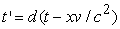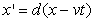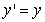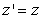Where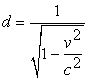2.1.1. First Relativity Claim

An object X with mass m in the reference frame B is not moving, but it is moving at speed v in the reference frame A.

The kinetic energy of X calculated in reference frame A is mv2/2.

In the inertial reference B, object X’s moving speed is zero. Therefore, the kinetic energy of X in reference frame B is zero.

The reference A and B draw different conclusions for the same experiment. One is mv2/2, the other is zero.

As stated in the Theory of Relativity,

The laws of physics are the same for all observers in uniform motion relative to one another (principle of relativity).

Both results are correct. Can we compare the two results? No, we can not, because mv2/2 is not zero.

Unfortunately, some interesting results of Relativity are derived by comparing results of two inertial references. Those results are: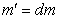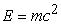The first Relativity claim is valid, but the Theory of Relativity use additional assumptions implicitly to get useful results.

2.1.2. Second Relativity Claim

Assuming that the second relativity claim is correct, then the third scenario for second Relativity claim, two moving light sources and two observers with different moving speed, should be valid.

It can be further simplified to two cases (Figure 3):

1. One of the receiver (reference) A (x, y, z) is moving at same speed with the light source, another B (x’, y’, z’) is moving toward the light source L.

2. The same as the above, except light source is L’ and L’ is not moving in B.

In case 1:In case 2: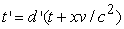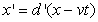The above two equations conclude that: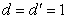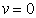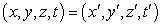The above results are not logical because,

(x, y, z, t) is not equal to (x’, y’, z’, t’)

v is not zero.

Therefore, as an elaborated claim of “constant one-way speed of light”, the second Relativity claim contradicts itself. Therefore, the one-way speed of light can not be constant.

3. Special Relativity Equations in Aether

The denial of “constant one-way speed of light” leads back to the theory of aether.

There are two moving parallel mirrors (Figure 4) A and B with a speed of v in the aether:

At time t in aether reference frame, the photon moves from A0 to B1. Then at 2t, it moves from B1 to A2. In the mean time, the two mirrors move as follow: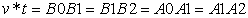From the moving mirror A perspective, A0, A1 and A2 are at the same spot on mirror A. From the moving mirror B’s perspective, B0, B1 and B2 are at the same spot on mirror B. The photon is bouncing back and force among three different points, A0, B1 and A2, in the aether reference, while the photon is bounced back and force between two points in the moving reference.

The moving reference uses the same light speed to measure space and time. Therefore, it has same light speed as aether reference and has its own time and space measurements lm and tm.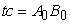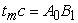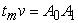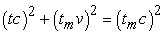Or,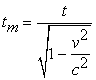As lm = tmc and l = tc: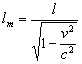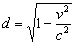The light can travel in the direction of the mirrors’ movement or opposite the direction of the mirrors’ movement.

When light travels along the moving direction: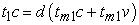When light travels opposite to the moving direction: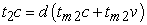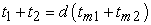To simply the above,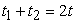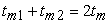The travelling time of light above is the two-way travelling time, which remains constant in all directions, while the one-way travelling time can differ when travelling parallel to the moving direction. In other words, the constant two-way speed of light is not only experimentally proven, but also logically sound.

The space/time expansion occurs in all directions with the same factor of d.

According to Planck equation, E=hc/L. In the moving reference, when the wavelength is 1, the aether reference wavelength is: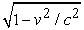Or,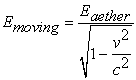(1)

Since,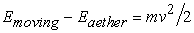(2)

From (1),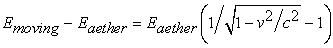(3)

When v is very small, (3) can be simplified as: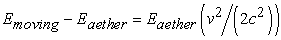(4)

Combine (3) and (4),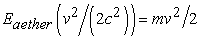Or,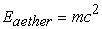(5)

4. Conclusions

We need to elucidate the first Relativity claim to be more specific:

The laws of physics are the same for all observers in uniform motion relative to one another (principle of relativity). The spacetime measurement is increased by factor of (1-(v/c)2)-1/2 relative to the aether reference when the speed of the inertial reference frame relative to aether is v. The two observers get same results when aether reference spacetime is used.

The Physics laws work fine as long as we measure test results based on our own spacetime in the same reference frame. But the aether reference is needed in order to get same test results.

The scientific community accepts the Theory of Relativity because when everything is moving, we all know that our tests’ conclusions are right based on our experiences. On the other hand, the Physicists get their Physics experiments’ results in one inertial reference frame. It is not easy to compare the results among different inertial reference frames without knowing the aether reference frame. Assuming that we are moving at a slower pace against the aether, using the Earth as a reference frame provides a good approximation for the mass of high speed particles in a particle accelerator.

The second Relativity claims:

The speed of light in a vacuum is the same for all observers, regardless of their relative motion or of the motion of the source of the light.

Since one-way speed of light is not constant, we can reformulate the claim as:

The two-way speed of light between two arbitrary locations in any inertial reference frame in a vacuum is the same.

The above claim limits the definition of constant light speed to constant two-way light speed.

The equations of Special Relativity are foundational for many physics theories. The findings on controversial Relativity Postulates shake the foundation. Fortunately, the Special Relativity equations can be derived without Postulates of Relativity and provide a better new foundation that is compatible with the existing physics theories.

We need to redefine the Postulates of Relativity with following new Postulates as our new foundation:

1. The laws of physics are the same for all observers in uniform motion relative to one another (principle of relativity). The spacetime measurement is increased by factor of (1-(v/c)2)-1/2 relative to aether reference when the speed of the inertial reference frame relative to aether is v. The two observers get same results when aether reference spacetime is used.

2. The two-way speed of light between two arbitrary locations in any inertial reference frame in a vacuum is the same.

References

  Einstein A. (1916 (translation 1920)), Relativity: The Special and General Theory, : H. Holt and Company.In article CrossRef  Planck, Max (1906), "The Measurements of Kaufmann on the Deflectability of ß-Rays in their Importance for the Dynamics of the Electrons", Physikalische Zeitschrift 7: 753-761In article  Miller, Arthur I. (1981), Albert Einstein's special theory of relativity. Emergence (1905) and early interpretation (1905-1911).In article  Will, Clifford M (August 1, 2010). "Relativity". Grolier Multimedia Encyclopedia. Retrieved 2010-08-01.In article  Will, Clifford M (August 1, 2010). "Space-Time Continuum". Grolier Multimedia Encyclopedia. Retrieved 2010-08-01.In article  Will, Clifford M (August 1, 2010). "Fitzgerald–Lorentz contraction". Grolier Multimedia Encyclopedia. Retrieved 2010-08-01.In article  Einstein, Albert (November 28, 1919). "Time, Space, and Gravitation". The Times.In article  Feynman, Richard Phillips; Morínigo, Fernando B.; Wagner, William; Pines, David; Hatfield, Brian (2002). Feynman Lectures on Gravitation. West view Press. p. 68.In article  Roberts, T; Schleif, S; Dlugosz, JM (ed.) (20 07). "What is the experimental basis of Special Relativity?". Usenet Physics FAQ. University of California, Riverside. Retrieved 2010-10-31.In article  Michael Tooley (2000). Time, tense, and causation. Oxford University Press. p. 350.In article CrossRef  Conventionality of Simultaneity entry by Allen Janis in the Stanford Encyclopedia of Philosophy, 2010.In article  Jong-Ping Hsu, Yuan-Zhong Zhang (2001). Lorentz and Poincaré Invariance: 100 Years of Relativity. World Scientific.In article  Will, C.M (2006). "Special Relativity: A Centenary Perspective". In T. Damour, O. Darrigol, B. Duplantier und V. Rivasseau. Poincare Seminar 2005. Basel: Birkhauser. pp. 33–58. arXiv:gr-qc/0504085.17th General Conference on Weights and Measures (1983), Resolution 1,Zhang (1997), p 24.In article  Will, Clifford M. (1992). "Clock synchronization and isotropy of the one-way speed of light". Physical Review D 45 (2): 403-411.In article CrossRef PubMed  Hafele, J. C.; Keating, R. E. (July 14, 1972). "Around-the-World Atomic Clocks: Predicted Relativistic Time Gains". Science 177 (4044): 166-168.In article CrossRef PubMed  C.O. Alley, in NASA Goddard Space Flight Center, Proc. of the 13th Ann. Precise Time and Time Interval (PTTI) Appl. and Planning Meeting, p. 687-724, 1981, available online.In article  Giulini, Domenico (2005). "Synchronization by slow clock-transport". Special Relativity: A First Encounter. 100 years since Einstein. Oxford University Press. ISBN 0191620866. Special Relativity: A First Encounter at Google Books.In article  Winnie, J. A. A. (1970). "Special Relativity without One Way Velocity Assumptions". Philosophy of Science 37: 81-99, 223-38.In article CrossRef  Debs, Talal A.; Redhead, Michael L. G. (1996). "The twin "paradox" and the conventionality of simultaneity". American Journal of Physics 64 (4): 384-392.In article CrossRef  Bailey et al (1977). "Measurements of relativistic time dilatation for positive and negative muons in a circular orbit". Nature 268 (5618): 301-305.In article CrossRef  Wesley C. Salmon (1977). "The Philosophical Significance of the One-Way Speed of Light". Noûs 11 (3): 253-292.In article CrossRef  Ohanian, Hans C. (2004). "The role of dynamics in the synchronization problem". American Journal of Physics 72 (2): 141-148.In article CrossRef  Ohanian, Hans C. (2005). "Reply to "Comment(s) on 'The role of dynamics in the synchronization problem'," by A. Macdonald and A. A. Martínez". American Journal of Physics 73 (5): 456-457.In article CrossRef  Martínez, Alberto A. (2005). "Conventions and inertial reference frames". American Journal of Physics 73 (5): 452-454.In article CrossRef  MacDonald, Alan (2004). "Comment on "The role of dynamics in the synchronization problem," by Hans C. Ohanian". American Journal of Physics 73 (5): 454-455.In article CrossRef  Iyer, Chandru; Prabhu, G. M. (2010). "A constructive formulation of the one-way speed of light". American Journal of Physics 78 (2): 195-203.In article CrossRef  Greaves, E. D.; Rodríguez, An Michel; Ruiz-Camacho, J. (2009), "A one-way speed of light experiment", American Journal of Physics 77 (10): 894-896.In article CrossRef  Finkelstein, J. (2009), "One-way speed of light?", American Journal of Physics 78 (8): 877.In article CrossRef  Roberts, Schleif (2006): Relativity FAQ, One-Way Tests of Light-Speed Isotropy.In article  Mansouri R., Sexl R.U. (1977). "A test theory of special relativity: II. First order tests". General. Relat. Gravit. 8 (7): 515-524.In article CrossRef  Krisher et al. (1990). "Test of the isotropy of the one-way speed of light using hydrogen-maser frequency standards". Physical Review D 42 (2): 731-734.In article CrossRef PubMed  Vessot et al (1980). "Test of relativistic gravitation with a space-borne hydrogen maser". Physical Review Letters 45 (29): 2081-2084.In article CrossRef  Riis et al (1988). "Test of the Isotropy of the speed of light using fast-beam laser spectroscopy". Physical Review Letters 60 (11): 81-84.In article CrossRef PubMed  Nelson et al. (1992). "Experimental comparison of time synchronization techniques by means of light signals and clock transport on the rotating earth". Proceedings of the 24th PTTI meeting 24: 87-104.In article PubMed  Wolf, Peter; Petit, Gérard (1997). "Satellite test of special relativity using the global positioning system". Physical Review A 56 (6): 4405-4409.In article CrossRef  Brecher, K. (1977), "Is the speed of light independent of the velocity of the source", Physical Review Letters 39: 1051-1054.In article CrossRef  Alväger, T.; Nilsson, A.; Kjellman, J. (1963), "A Direct Terrestrial Test of the Second Postulate of Special Relativity", Nature 197 (4873): 1191.In article CrossRef  Amelino-Camelia, G (2009). "Astrophysics: Burst of support for relativity". Lay summary – Nature (19 November 2009). Nature 462 (7271): 291-292.In article CrossRef PubMed  Laurent et al. (2011). "Constraints on Lorentz Invariance Violation using integral/IBIS observations of GRB041219A". Physical Review D 83 (12): 121301.In article CrossRef  Kostelecký, V. Alan; Mewes, Matthew (2002). "Signals for Lorentz violation in electrodynamics". Physical Review D 66 (5): 056005.In article CrossRef